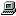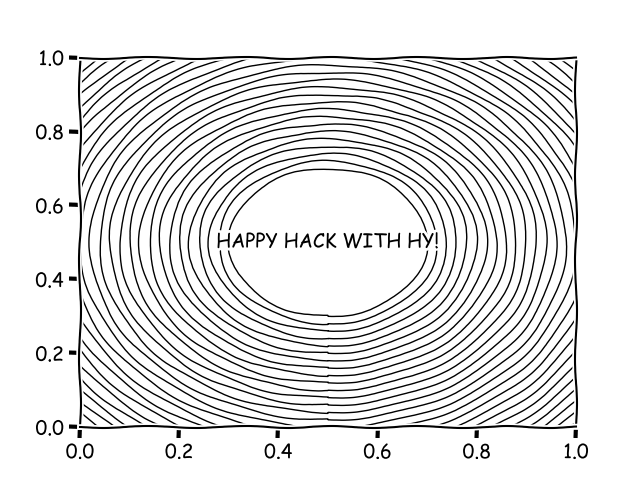# NaNg's blog

25 object(s)# Python生态下的Lisp方言## 环境配置

``````\$ pip install git+https://github.com/hylang/hy.git
# 注意 这里不要直接 pip install hy 这样从pypi直接安装，有坑...我已经踩过了
``````

## 动手写

``````(import [matplotlib [pyplot :as plt]])
(import time)

(.xkcd plt)
(setv (, fig, ax) (.subplots plt))

(defn draw-circle [r0 r-max r-step]
(setv r r0)
(if (< r r-max) (do (-> (.Circle plt (, 0.5 0.5) r :color "black" :fill False) (ax.add-artist))
(setv r (+ r r-step))
(draw-circle r r-max r-step))))

(draw-circle 0.2 1 0.02)
(.text plt 0.5 0.5 (.upper "happy hack with hy!") :va "center" :ha "center")
(.show plt)
``````### 伪装成Lisp的Python

``````;字符串
;Hy的字符机制和Python3是一致的，统一使用Unicode，摒弃了Python2的错误
;Hy的字符串有两种写法,第一种在Hy中称为Symbol,语法是在字母前加一个单引号：
=>'hello
u"hello"
=> '你好
u'\u4f60\u597d'

;第二种是双引号括起来的字符串，可以表示多行字符类似于Python中的三引号
=> "[Q]:what is hy?
... [A]:A dialect of Lisp
... that's embedded in Python"
u"[Q]:what is hy?\n[A]:A dialect of Lisp \nthat's embedded in Python"

;列表
;Python中最常用的数据结构在Hy中还是用方括号括起来只不过不用写中间的逗号了
=> [1 2 3 4]
[1L, 2L, 3L, 4L]
=> (def data ["panty" "stocking" "garterbelt"])
=> (get data 1)
u"stocking"

;元组
;用S表达式来表达，操作符是逗号
=> (, "hello" "hy")
(u'hello', u'hy')

;字典
=> {'Sunday '星期天 'Saturday '星期天}
{u'Sunday': u'\u661f\u671f\u5929', u'Saturday': u'\u661f\u671f\u5929'}
=> (print (get _ 'Sunday))

``````

``````;赋值可用 def 或者 setv 但一般def用于定义全局变量,setv用于定义局部变量
=> (def n 41)
=> (print n)
41
=> (setv n (inc n))
=> (print n)
42
;除了这些，还有Lisp中的let，可以用来显式地创建词法作用域
=> (let [n 'nana
...      q 'haqi]
...     (print (+ n " " q)))
nana haqi
=> n
42L
=> q
Traceback (most recent call last):
File "<input type="text" />", line 1, in
NameError: name 'q' is not defined

;条件语句
=> (print (if (< n 0.0) "negative" ... (= n 0.0) "zero" ... (> n 0.0) "positive"
...            "not a number"))
positive
;或者用cond
=> (print (cond [(< n 0.0) "negative"] ... [(= n 0.0) "zero"] ... [(> n 0.0) "positive"]
...              [True "not a number"]))
positive
;for循环
=> (for [x (range 3)] (print x))
0
1
2
;while循环
(while True (print 'hy))
hy
hy
hy
...

;函数定义，没有显式的return，最后一条表达式的求值结果就是返回值
=> (defn fizzbuzz [i]
...   (cond [(= 0 (% i 15)) 'FizzBuzz]
...         [(= 0 (% i 3)) 'Fizz]
...         [(= 0 (% i 5)) 'Buzz]
...         [True i]))
;函数调用
=> (fizzbuzz 15)
u'FizzBuzz'

``````

``````;列表推导
=> (list-comp (** x 2) [x (range 10)])
[0L, 1L, 4L, 9L, 16L, 25L, 36L, 49L, 64L, 81L]
;带条件后缀的列表推导
=> (list-comp (** x 2) [x (range 10)] (odd? x))
[1L, 9L, 25L, 49L, 81L]
;字典推导
=> (dict-comp x (* x 2) [x (range 10)] (odd? x))
{1: 2, 3: 6, 9: 18, 5: 10, 7: 14}
;genexpr ；类似于列表推导但产生的是一个generator
=> (def filtered (genexpr x [x (range 10)] (even? x)))
=> (list filtered)
[0, 2, 4, 6, 8]

;with
=> (with [f (open "./test.txt")] (print (.read f)))
first line
second line

;生成器函数(yield)
=> (defn fib []
...   (setv (, a b) (, 0 1))
...   (while True
...     (yield a)
...     (setv (, a b) (, b (+ a b)))))
=> (list (take 10 (fib)))
[0L, 1L, 1L, 2L, 3L, 5L, 8L, 13L, 21L, 34L]
``````

``````;这样定义类
=> (defclass FooBar [object]
...   "Yet Another Example Class"
...
...   (defn --init-- [self x]
...     (setv self.x x))
...
...   (defn get-x [self]
...     "Return our copy of x"
...     self.x))
;创建对象
=> (def foobar (FooBar 42))
=> foobar

=> foobar.x
42L
;方法调用
=> (foobar.get-x)
42L
;你也可以用前缀表达式调用类方法，并且更建议这么做
=> (.get-x foobar)
42L
``````

## 函数式编程

``````;首先Python中的 map、reduce、filter 以及 lambda 这些函数式编程的基本组件在Hy中还是一样的
;lambda算子(匿名函数) 和可以使用fn或者lambda来表示
=> (fn [x] (+ x 1))
=> (lambda [x] (+ x 1))
<function <lambda> at 0x7f9b61fe1de8>
=> (_ 1)
2L

;Hy中的map、filter和Python3是一致的，返回的是一个可迭代的延迟求值的对象，而非Python2那样直接返回列表
;map
=> (map (fn [x] (* x x)) (range 1 11))

=> (list _) ;这时把它转换成list的时候才会对map进行求值
[1, 4, 9, 16, 25, 36, 49, 64, 81, 100]
;filter
=> (filter odd? (range 1 11))

=> (list _)
[1, 3, 5, 7, 9]
;reduce
=> (reduce (fn [a b] (* a b)) (range 1 11)) ;从1开始累乘到10
3628800
``````
##### 尾递归优化``````(require [hy.contrib.loop [loop]]) ;导入外部宏

(defn factorial [n]
(loop [[i n] [acc 1]]
;通过loop创建递归的开始点，并初始化递归参数
(if (zero? i)
acc
;通过recur进入下一轮递归，如果recur不写在结尾位置就会报错
(recur (dec i) (* acc i)))))

(factorial 1000)
``````
##### 管道

``````=> (filter odd? (map inc (map (fn [x] (* x x)) (range 100))))

=> (list _)
[1L, 5L, 17L, 37L, 65L, 101L, 145L, 197L, 257L, 325L, 401L, ...]
``````

``````=> (->> (range 100) (map (fn [x] (* x x))) (map inc) (filter odd?))

=> (list _)
[1L, 5L, 17L, 37L, 65L, 101L, 145L, 197L, 257L, 325L, 401L, ...]
``````

``````=> (import [sh [cat grep wc]]) ;需要事先安装sh模块: pip install sh
=> (-> (cat "/usr/share/dict/words") (grep "-E" "^hy") (wc "-l"))
210
``````

``````(require [hy.contrib.anaphoric [ap-pipe]])

=> (ap-pipe 3 (+ it 1) (/ 5 it))
1.25
=> (ap-pipe [4 5 6 7] (list (rest it)) (len it))
3
``````

``````=> (doto [] (.append 1) (.append 2) .reverse)
[2L, 1L]
``````

## 来自Lisp的力量:宏``````(require [hy.contrib.loop [loop]])
(import time)
(import sys)

(.setrecursionlimit sys 1000) ;放宽递归深度限制

(defn fib-recur [n] ;递归的写法
(if (in n '(0 1))
(long n)
(+ (fib-recur (- n 1)) (fib-recur (- n 2)))))

(defn fib-iter [n &optional [a 0] [b 1]] ;迭代的写法，但形式上还是递归的
(if (zero? n)
a
(fib-iter (dec n) b (+ a b))))

(defn fib-tco [n] ;使用尾调用优化的写法
(loop [[i n] [a 0] [b 1]]
(if (zero? i)
a
(recur (dec i) b (+ a b)))))

(defn fib-gen [] ;使用生成器的写法
(setv (, a b) (, 0 1))
(while True
(yield a)
(setv (, a b) (, b (+ a b)))))
``````

``````(def s (.time time))
(-> (fib-iter 500) print)
(-> (- (.time time) s) print)
``````

``````(defmacro timeit [code &optional [label "code"]]
`(do
(setv s (.time time))
~code
(print (.format "{l} run cost {t}s.\n" :l ~label :t (- (.time time) s)))))
``````

``````;递归写法的fib计算实在太慢，这里就不考虑它了...
(timeit (-> (fib-iter 500) print) 'fib-iter)
(timeit (-> (fib-tco 500) print) 'fib-tco)
(timeit (-> (nth (fib-gen) 500) print) 'fib-gen)
;运行结果：
;139423224561697880139724382870407283950070256587697307264108962948325571622863290691557658876222521294125
;fib_iter run cost 0.000308990478516s.
;
;139423224561697880139724382870407283950070256587697307264108962948325571622863290691557658876222521294125
;fib_tco run cost 0.000863075256348s.
;
;139423224561697880139724382870407283950070256587697307264108962948325571622863290691557658876222521294125
;fib_gen run cost 0.000124216079712s.
``````

``````(defreader t [code]
`(do
(setv s (.time time))
~code
(print (.format "code run cost {t}s.\n" :t (- (.time time) s)))))

#t(-> (nth (fib-gen) 500) print)
;结果:
;139423224561697880139724382870407283950070256587697307264108962948325571622863290691557658876222521294125
;code run cost 0.000133037567139s.
``````

## 迈向实用：与Python交互

Hy根植于Python生态，可以与Python之间无缝衔接，使用Python的库只需要import就行，与Python中存在的那几种import方式是相对应的，比如:

``````(import requests) ;相当于 import requests
(import [Bio [Seq Align]]) ;from Bio import Seq, Align
(import [Bio [*]]) ;from Bio import *
(import [matplotlib [pyplot :as plt]]) ;from matplotlib import pyplot as plt
``````

``````>>> import hy # 在导入Hy模块前需要先import hy
>>> from fib_bench import *
>>> [fib_iter(i) for i in range(10)]
[0L, 1L, 1L, 2L, 3L, 5L, 8L, 13L, 21L, 34L]
``````